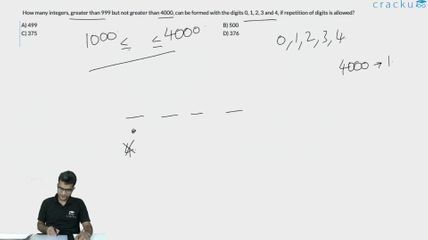Question 10

# How many integers, greater than 999 but not greater than 4000, can be formed with the digits 0, 1, 2, 3 and 4, if repetition of digits is allowed?

Solution

We have to essentially look at numbers between 1000 and 4000 (including both).

The first digit can be either 1 or 2 or 3.

The second digit can be any of the five numbers.

The third digit can be any of the five numbers.

The fourth digit can also be any of the five numbers.

So, total is 3*5*5*5 = 375.

However, we have ignored the number 4000 in this calculation and hence the total is 375+1=376

### View Video Solution